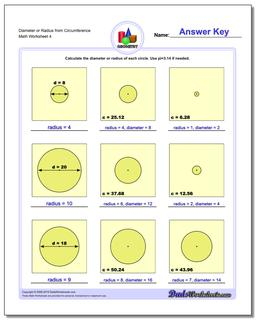# Math Worksheets: Basic Geometry: Basic Geometry: Diameter or Radius from Circumference (Fourth Worksheet)## Diameter or Radius from Circumference (Fourth Worksheet)

PropertyValue
DescriptionDiameter or Radius from Circumference: Worksheets to calculate radius from diameter, diameter from radius, or radius and diameter from area or circumference. (Fourth Worksheet)
Resource TypeWorksheet